Courses

# Asymptotic Worst Case Time & Space Complexity MCQ - 4

## 20 Questions MCQ Test GATE Computer Science Engineering(CSE) 2022 Mock Test Series | Asymptotic Worst Case Time & Space Complexity MCQ - 4

Description
This mock test of Asymptotic Worst Case Time & Space Complexity MCQ - 4 for Computer Science Engineering (CSE) helps you for every Computer Science Engineering (CSE) entrance exam. This contains 20 Multiple Choice Questions for Computer Science Engineering (CSE) Asymptotic Worst Case Time & Space Complexity MCQ - 4 (mcq) to study with solutions a complete question bank. The solved questions answers in this Asymptotic Worst Case Time & Space Complexity MCQ - 4 quiz give you a good mix of easy questions and tough questions. Computer Science Engineering (CSE) students definitely take this Asymptotic Worst Case Time & Space Complexity MCQ - 4 exercise for a better result in the exam. You can find other Asymptotic Worst Case Time & Space Complexity MCQ - 4 extra questions, long questions & short questions for Computer Science Engineering (CSE) on EduRev as well by searching above.
QUESTION: 1

### Which of the following is TRUE?

Solution:

AVL tree is a balanced tree.
AVL tree's time complexity of searching = θ(logn)
But a binary search tree, may be skewed tree, so in worst case BST searching time = θ(n)

QUESTION: 2

Solution:
QUESTION: 3

### If we use Radix Sort to sort n integers in the range (nk/2,nk], for some k>0 which is independent of n, the time taken would be?

Solution:

Radix sort time complexity = O(wn)
for n keys of word size= w
=>w = log(nk
O(wn)=O(klogn.n)
=> kO(nlogn)

QUESTION: 4

The auxiliary space of insertion sort is O(1), what does O(1) mean?

Solution:

The term O(1) states that the space required by the insertion sort is constant i.e., space required doesn't depend on input.

QUESTION: 5

What is the value of following recurrence.

T(n) = T(n/4) + T(n/2) + cn^2
T(1) = c
T(0) = 0

Q. Where c is a positive constant

Solution:

Following is the initial recursion tree for the given recurrence relation.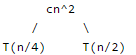If we further break down the expression T(n/4) and T(n/2), we get following recursion tree.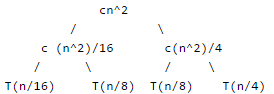Breaking down further gives us following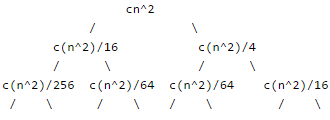To know the value of T(n), we need to calculate sum of tree nodes level by level. If we sum the above tree level by level, we get the following series T(n) = c(n^2 + 5(n^2)/16 + 25(n^2)/256) + .... The above series is geometrical progression with ratio 5/16 To get an upper bound, we can sum the above series for infinite terms. We get the sum as (n^2) / (1 - 5/16) which is O(n^2)

QUESTION: 6

What is the value of following recurrence. T(n) = 5T(n/5) +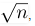, T(1) = 1, T(0) = 0

Solution:

The given solution can be solved using Master Method. It falls in Case 1.

QUESTION: 7

What is the worst case time complexity of following implementation of subset sum problem.

// Returns true if there is a subset of set[] with sun equal to given sum

bool isSubsetSum(int set[], int n, int sum)

{

// Base Cases

if (sum == 0)

return true;

if (n == 0 && sum != 0)

return false;

// If last element is greater than sum, then ignore it

if (set[n-1] > sum)

return isSubsetSum(set, n-1, sum);

/* else, check if sum can be obtained by any of the following

(a) including the last element

(b) excluding the last element   */

return isSubsetSum(set, n-1, sum) ||

isSubsetSum(set, n-1, sum-set[n-1]);

}

Solution:

Following is the recurrence for given implementation of subset sum problem T(n) = 2T(n-1) + C1 T(0) = C1 Where C1 and C2 are some machine specific constants. The solution of recurrence is O(2^n) We can see it with the help of recurrence tree method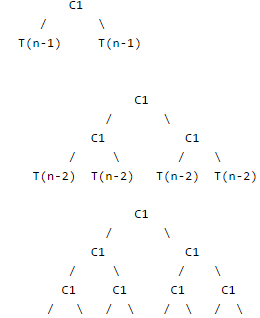If we sum the above tree level by level, we get the following series T(n) = C1 + 2C1 + 4C1 + 8C1 + ...
The above series is Geometrical progression and there will be n terms in it.
So T(n) = O(2^n)

QUESTION: 8

Suppose T(n) = 2T(n/2) + n, T(0) = T(1) = 1 Which one of the following is false.
a) T(n) = O(n^2)
b) T(n) = θ(nLogn)
c) T(n) = Ω(n^2)
d) T(n) = O(nLogn)

Solution:
QUESTION: 9

Consider the following recurrence: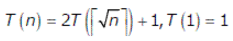Q. Which one of the following is true?

Solution:

This question can be solved by first change of variable and then Master Method.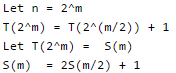Above expression is a binary tree traversal recursion whose time complexity is θ(m). You can also prove using Master theorem.

S(m) = θ (m)
= θ (log n)

/* since n = 2^m */

Now, let us go back to the original recursive function T(n)

T(n) = T(2^m) = s(m)

=  θ (log n)

QUESTION: 10

The running time of an algorithm is represented by the following recurrence relation: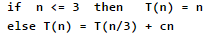Q. Which one of the following represents the time complexity of the algorithm?

Solution: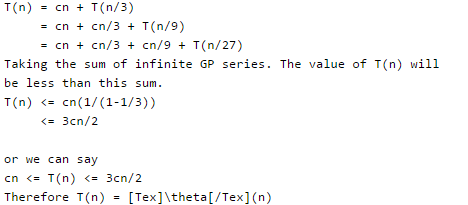This can also be solved using Master Theorem for solving recurrences. The given expression lies in Case 3 of the theorem.

QUESTION: 11

The running time of the following algorithm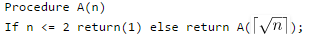is best described by

Solution:
QUESTION: 12

What is the time complexity of the following recursive function: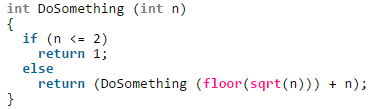Solution:

Recursive relation for the DoSomething() is

T(n) = T(√n) + C1 if n > 2

We have ignored the floor() part as it doesn't matter here if it's a floor or ceiling.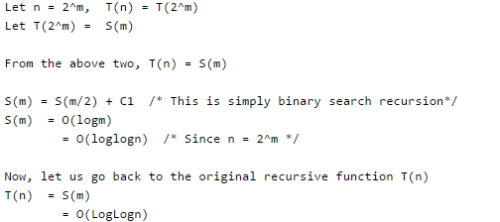QUESTION: 13

The time complexity of the following C function is (assume n > 0

int recursive (int n)
{
if(n == 1)
return (1);
else
return (recursive (n-1) + recursive (n-1));
}

Solution:

Recursive expression for the above program will be.

T(n) = 2T(n-1) + c
T(1) = c1.

Let us solve it.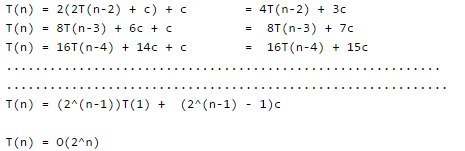QUESTION: 14

Consider the following recurrence T(n) = 3T(n/5) + lgn * lgn What is the value of T(n)?

Solution:

By Case 1 of the Master Method, we have T(n) = θ(n ^ (log5(3))). [^ is for power]

QUESTION: 15

Consider the following recurrence.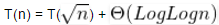Q. What is the value of recurrence?

Solution:

Change of variables: let m = lg n. Recurrence becomes S(m) = S(m/2) + θ(lgm). Case 2 of master’s theorem applies, so T(n) = θ( (log Log n) ^ 2).

QUESTION: 16

Which one of the following correctly determines the solution of the recurrence relation with T(1) = 1?

T(n) = 2T(n/2) + Logn

Solution: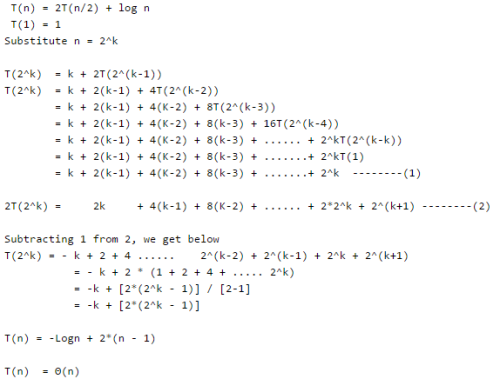QUESTION: 17

Consider the following recurrence relation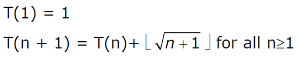The value of T(m2) for m ≥ 1 is

Solution: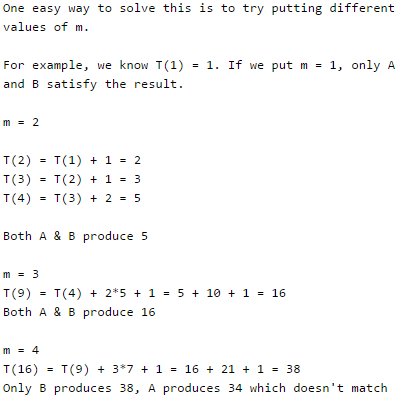QUESTION: 18

The solution to the recurrence equation T(2k) = 3 T(2k-1) + 1, T (1) = 1, is:

Solution:

We have T (2k) = 3  T (2k-1) + 1 = 32  T (2k-2) + 1 + 3 = 33  T (2k-3) + 1 + 3 + 9 . . . (k steps of recursion (recursion depth)) = 3k  T (2k-k) + (1 + 3 + 9 + 27 + ... + 3k-1) = 3k + (( 3k - 1)/2) = ((2 * 3k) + 3k - 1)/2 = ((3 * 3k) - 1)/2 = (3k+1 - 1)/2 Hence, B is the correct choice.

QUESTION: 19

Select the correct asymptotic complexity of an algorithm with runtime T(n, n) where

T(x, c) = Θ(x) for c <= 2,
T(c, y) = Θ(y) for c <= 2, and
T(x, y) = Θ(x+y) + T(x/2, y/2)

Solution:

The recurrence is

T(x, y) = Θ(x+y) + T(x/2, y/2)

It can be written as below.

T(x, y) = Θ(x+y) + Θ((x+y)/2) + Θ((x+y)/4) + Θ((x+y)/8) .....
T(x, y) = Θ((x+y) + (x+y)/2 + (x+y)/4 + (x+y)/8 ..... )

The above expression forms a geometric series with ratio as 2 and starting element as (x+y)/2 T(x, y) is upper bounded by Θ(x+y) as sum of infinite series is 2(x+y). It is lower bounded by (x+y)

QUESTION: 20

Let f(n) = n and g(n) = n(1+sin n), where n is a positive integer. Which of the following statements is/are correct?

I. f(n) = O(g(n))
II. f(n) = Ω(g(n))

Solution:

The value of sine function varies from -1 to 1.

For sin = -1 or any other negative value, I becomes false.

For sin = 1 or any other negative value, II becomes false